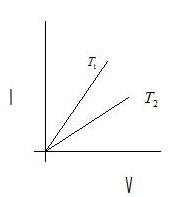# Current electricity test series

(A) Assertion reason type question.

(a) If both assertion and reason are true and reason is correct explaination of assertion.
(b) If both assertion and reason are true and reason is not correct explaination of assertion.
(c) Assertion is true but reason is false.
(d) both assertion and reason are false.

(1)
Assertion:Wire carrying current is not charged.
Reason:It is because , at any instant number of electrons leaving wire is sometimes equal to the number of electrons flowing in from the battery.

(2)
Assertion:Drift velocity of electrons decreases on increasing temperature of the conductor.
Reason:It is because, on increasing temperature of a conductor , the value of resistivity of it's material increases.

(3)
Assertion:Resistors are connected in series combination in order to increase the resistance of the circuit.
Reason:In series combination potential difference across any resistor is proportional to it's resistance.

(4)
Assertion:A potentiometer measures the potential difference more accurately then a voltmeter.
Reason:Because it has a wire of high resistance and draws a heavy current from external circuit.

(B) Multiple choice questions with only one answer

(5) A constant voltage is applied between the two ends of a uniform metalic wire and some heat is developed in it . The heat developed is doubled if,
(a) both length and radius of wire are halved
(b) both length and radius of wire are doubled
(c) radius of wire is doubled
(d) length of wire is doubled

(6) Kirchoff's first law of electric circuits is based on the law of conservation of
(a) only on mass
(b) only on charge
(c) on charge as well as on energy
(d) on charge as well as on mass

(7)The temperature coefficent of resistance of wire is 0.00125°C-1. At 300K, it's resistance is 1 ohm. The resistance of wire would be 2 ohm at
(a) 1154K
(b) 1127K
(c) 1167K
(d) 1176K

(8) If the length of the potentiometer wire is increased, then the accuracy in determination of null point
(a) will increase
(b) will decrease
(c) will remain unaffected
(d) can not be decided , untill emf of auxiliary battery is known

(9) Each of the resistance in the network shown in the figure is equal to R. The resistance between terminal A and B is(a) R
(b) 5R
(c) 3R
(d) 6R

(10)The current voltage graph for a given metallic wire at two different temperatures T1 and T2 are shown in the fig. which one of the following option is true(a) T1 = T2
(b) T1 < T2
(c) T1 > T2
(d) none of the above

Solutions
1.c
2.a
3.b
4.c
5.b
6.b
7.b
8.a
9.a
10.b